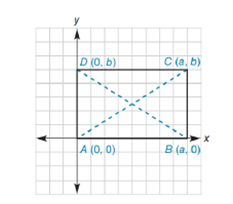Chapter 10.1, Problem 30EElementary Geometry For College St...

7th Edition
Alexander + 2 others
ISBN: 9781337614085

Solutions

Chapter
SectionElementary Geometry For College St...

7th Edition
Alexander + 2 others
ISBN: 9781337614085
Textbook Problem

The rectangle whose vertices are A 0, 0 ,   B a ,   0 ,   C a ,   b , and D ( 0 ,   b ) is shown. Use the Distance Formula to draw a conclusion concerning the length of the diagonals A C - and B D - .To determine

To find:

The length of the diagonals AC- and BD- of the rectangle whose vertices are A0, 0, Ba, 0, Ca, b, and D(0, b).

Explanation

Given vertices are,

A0, 0, Ba, 0, Ca, b, and D(0, b).

Sketch the points in the graph and draw the diagonals AC- and BD- as shown in below graph,

Length of the diagonals AC- and BD- can be determined using distance formula,

The distance between the two points (x1, y1) and (x2, y2) as below,

d=(x2-x1)2+(y2-y1)2

Coordinates of A(0, 0), C(a, b)

Length of AC=(a-0)2+(b-0)2

Leng

Still sussing out bartleby?

Check out a sample textbook solution.

See a sample solution

The Solution to Your Study Problems

Bartleby provides explanations to thousands of textbook problems written by our experts, many with advanced degrees!

Get Started